The goal of this challenge is to find all instances of dolphins in a picture and then color pixes of each dolphin with a unique color.

## Model

Here is an example of how to create a model for instance segmentation:

def get_instance_segmentation_model(hidden_layer_size, box_score_thresh=0.5):
# our dataset has two classes only - background and dolphin
num_classes = 2

# load an instance segmentation model pre-trained on COCO
pretrained=True,
box_score_thresh=box_score_thresh,
)

# get the number of input features for the classifier
# replace the pre-trained head with a new one

# now get the number of input features for the mask classifier

dim_reduced=hidden_layer_size,
num_classes=num_classes
)

return model

# get the model using our helper function
model = get_instance_segmentation_model(hidden_layer_size=256)

# move model to the right device
model.to(device)

# construct an optimizer
params = [p for p in model.parameters() if p.requires_grad]
optimizer = torch.optim.SGD(params, lr=0.005, momentum=0.9, weight_decay=0.0005)

# and a learning rate scheduler which decreases the learning rate by
# 10x every 3 epochs
lr_scheduler = torch.optim.lr_scheduler.StepLR(optimizer, step_size=10, gamma=0.1)


#### train_one_epoch[source]

train_one_epoch(model, optimizer, data_loader, device, epoch, print_freq=10)

Trains one epoch of the model. Copied from the reference implementation from https://github.com/pytorch/vision.git.

For training the model, you can use train_one_epoch as follows:

This is how to use the training function in training loop:

if Path("saved_models").exists():
saved_model_path = Path("./saved_models/model.pt")
else:
saved_model_path = Path("./notebooks/saved_models/model.pt")

if saved_model_path.exists():
num_epochs=1
else:
num_epochs = 20


for epoch in range(num_epochs):
# train for one epoch, printing every 20 iterations
train_one_epoch(model, optimizer, data_loader, device, epoch, print_freq=20)

# update the learning rate
lr_scheduler.step()


#### show_prediction[source]

show_prediction(model, img:tensor([]), score_threshold:float=0.5, width:int=820)

Show a single prediction by the model

Show predictions on a single input image:

# pick one image from the test set

show_prediction(model, img)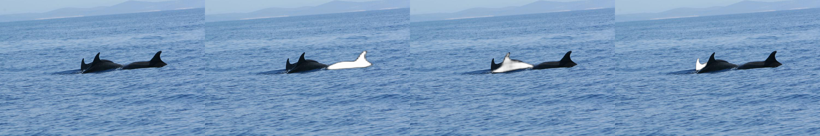We can also show predictions for the whole or for a subset of the dataset from a dataloader object:

#### show_predictions[source]

show_predictions(model, data_loader=None, dataset=None, n=None, score_threshold=0.5, iou_df=None, width=820)

Show at most n predictions for examples in a given data loader.

Shows predictions for the first two elements in the data loader:

show_predictions(model, data_loader=data_loader_test, n=2, score_threshold=0.5)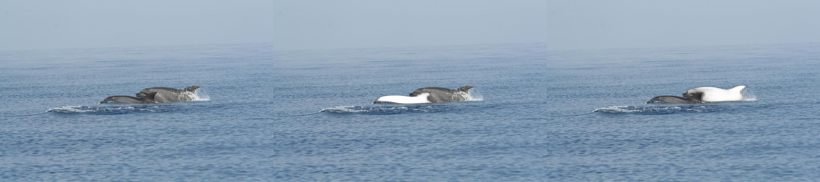## Metrics

_, masks = get_true_and_predicted_masks(model, data_loader_test.dataset, 0.5)

print(f'We have {masks["true"].shape} dolphins on the photo, total of {masks["predicted"].shape} are predicted with score higher than 0.5')

show_prediction(model, img)

We have 3 dolphins on the photo, total of 3 are predicted with score higher than 0.5### Metric explanation

For evaluating instance segmentation results, we would be using a metric called Intersection over Union or IoU. The IoU metric is a method to quantify the percent overlap between the ground-truth (or target) mask and the predicted (output) mask. i. e., the IoU metric measures the number of pixels common between the ground-truth and prediction masks divided by the total number of pixels present across both masks and is mathematically represented as:

$$IoU = \frac{{target \cap prediction}}{{target \cup prediction}}$$

As a visual example, let's suppose we're tasked with calculating the IoU score of a prediction mask (colored yellow), given the ground truth labeled mask (colored blue). The intersection (A∩B) is comprised of the pixels found in both the prediction mask and the ground truth mask (denoted in green color), whereas the union (A∪B) is simply comprised of all pixels found in either the prediction or target mask.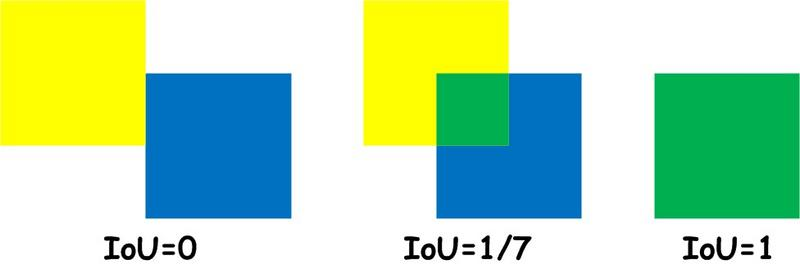Image credits: link

As can be seen from the above example, more the intersection or overlap between the ground truth and the predicted mask, greater is the IoU metric value. The maximum IoU metric value of 1 is obtained when both the predicted mask and ground-truth mask overlap perfectly and the minimum value of 0 is obtained when there is absolutely no overlap.

#### iou_metric_mask_pair[source]

iou_metric_mask_pair(binary_segmentation:array, binary_gt_label:array)

Compute the IOU between two binary segmentation (typically one ground truth and a predicted one). Input: binary_segmentation: binary 2D numpy array representing the region of interest as segmented by the algorithm binary_gt_label: binary 2D numpy array representing the region of interest as provided in the database Output: IOU: IOU between the segmentation and the ground truth

The above explanation is for a pair of single ground-truth or true mask and single predicted mask. Intersection over union metrics (IOU) for a pair of true and predicted masks can be calculated as follows:

img, masks = get_true_and_predicted_masks(model, data_loader_test.dataset)

# calculate the metrics
)

0.009040863480619882

In the instance segmentation task, a single image might predict multiple instance segmentation masks and the output prediction masks might not necessarily be in the same order as the ground-truth masks, i.e., the ordering of true and predicted masks can be and usually is different. In the example bellow, we have three true or ground truth masks but four predicted masks with score larger than 0.5:

#### iou_metric_matrix_of_example[source]

iou_metric_matrix_of_example(model:MaskRCNN, example:Tuple[Tensor, Dict[str, Tensor]], score_threshold:float=0.5)

metrics = iou_metric_matrix_of_example(model, data_loader_test.dataset, 0.5)

cm = sns.light_palette("lightblue", as_cmap=True)

df = pd.DataFrame(metrics)

0 1 2
0 0.009041 0.000000 0.699953
1 0.609545 0.168043 0.006262
2 0.031733 0.531035 0.000000

For a single input image, which contains multiple prediction masks and ground-truth masks (since there can be more than one dophin in the image), we first calculate the IOU metric for all the predicted and gound-truth pairs .

In the example above, we have three dolphins with three true masks, while the model predicted four masks. This is why the matrix above has four rows (corresponding to predictions) and three columns (corresponding to ground truth). The first mask predicted by the model is represented by the first row (row 0). As we can see, the best fitting is with the third true mask (column 2). The second predicted mask is represented with the second row (row 1) and the best fit is with the first true mask (column 1) and so on. The last row is an extra prediction.

Thus for a single input image, we calculate the IOU metric in such a way that the total IOU score for the image is maximized. That is, in the above example the IOU metric for the first predicted mask is taken as 0.691147 and 0.586046, 0.514863, 0.000 for the second, third and fourth respectively and take the mean of the four IOU metric values to obtain the IOU metric for single example image. The last one is an extra incorrect prediction and hence it is assigned the value of 0.000.

We repeat the above for all the images in the dataset and take the mean of the IOU values to obtain the IOU metric value for the entire dataset

#### largest_values_in_row_colums[source]

largest_values_in_row_colums(xs:array)

Approximates the largest value in each row/column.

largest_values_in_row_colums(metrics)

[0.6999534555127418, 0.6095453855961998, 0.531034752278878]

#### iou_metric_example[source]

iou_metric_example(model:MaskRCNN, example:Tuple[Tensor, Dict[str, Tensor]], score_threshold:float=0.5)

Finally, we can get IOU metrics for the whole image:

metric = iou_metric_example(model, data_loader_test.dataset, 0.5)

print(f"Average IOU metric on given example is {metric:.3f}")

Average IOU metric on given example is 0.300


#### iou_metric[source]

iou_metric(model:MaskRCNN, dataset:Dataset, score_threshold:float=0.5)

Calculate IOU metric on the whole dataloader

iou, iou_df = iou_metric(model, data_loader_test.dataset)


CPU times: user 10.1 s, sys: 7.85 ms, total: 10.1 s
Wall time: 6.59 s

paths iou
22 data/dolphins_200_train_val/Val/JPEGImages/140830_47_1_0471.jpg 0.217136
7 data/dolphins_200_train_val/Val/JPEGImages/140701_6_1_0025.jpg 0.235162
36 data/dolphins_200_train_val/Val/JPEGImages/190706_17_1_0215.jpg 0.238868
34 data/dolphins_200_train_val/Val/JPEGImages/190627_12_2_0125.jpg 0.248578
15 data/dolphins_200_train_val/Val/JPEGImages/140724_16_2_0385.jpg 0.293751
4 data/dolphins_200_train_val/Val/JPEGImages/140426_3_1_0130.jpg 0.300476
17 data/dolphins_200_train_val/Val/JPEGImages/140810_31_1_0054.jpg 0.301728
38 data/dolphins_200_train_val/Val/JPEGImages/190819_43_1_0234.jpg 0.303083
32 data/dolphins_200_train_val/Val/JPEGImages/170829_34_1_0103.jpg 0.308663
26 data/dolphins_200_train_val/Val/JPEGImages/150728_83_1_1180.jpg 0.328465
21 data/dolphins_200_train_val/Val/JPEGImages/140810_38_1_0263.jpg 0.336140
29 data/dolphins_200_train_val/Val/JPEGImages/170723_19_1_0055.jpg 0.336448
6 data/dolphins_200_train_val/Val/JPEGImages/140701_5_1_0043.jpg 0.342803
27 data/dolphins_200_train_val/Val/JPEGImages/170612_1_1_0110.jpg 0.361572
10 data/dolphins_200_train_val/Val/JPEGImages/140720_15_1_0424.jpg 0.365243
33 data/dolphins_200_train_val/Val/JPEGImages/190611_4_1_0489.jpg 0.367608
9 data/dolphins_200_train_val/Val/JPEGImages/140717_12_1_0407.jpg 0.421964
8 data/dolphins_200_train_val/Val/JPEGImages/140704_9_1_0058.jpg 0.422771
35 data/dolphins_200_train_val/Val/JPEGImages/190701_14_1_0067.jpg 0.430506
11 data/dolphins_200_train_val/Val/JPEGImages/140720_15_1_0463.jpg 0.445086
5 data/dolphins_200_train_val/Val/JPEGImages/140426_4_1_0117.jpg 0.446499
16 data/dolphins_200_train_val/Val/JPEGImages/140728_20_1_0698.jpg 0.447267
25 data/dolphins_200_train_val/Val/JPEGImages/150724_78_1_0667.jpg 0.465779
2 data/dolphins_200_train_val/Val/JPEGImages/070828_20_1_0060.jpg 0.469470
37 data/dolphins_200_train_val/Val/JPEGImages/190819_43_1_0108.jpg 0.474755
19 data/dolphins_200_train_val/Val/JPEGImages/140810_33_1_0254.jpg 0.507112
31 data/dolphins_200_train_val/Val/JPEGImages/170808_27_1_0287.jpg 0.544885
13 data/dolphins_200_train_val/Val/JPEGImages/140724_16_1_0003.jpg 0.562608
30 data/dolphins_200_train_val/Val/JPEGImages/170723_19_1_0094.jpg 0.603903
0 data/dolphins_200_train_val/Val/JPEGImages/070729_11_2_0026.jpg 0.613511
28 data/dolphins_200_train_val/Val/JPEGImages/170612_1_1_0424.jpg 0.615975
20 data/dolphins_200_train_val/Val/JPEGImages/140810_35_3_0074.jpg 0.617149
1 data/dolphins_200_train_val/Val/JPEGImages/070730_13_2_0100.jpg 0.632938
3 data/dolphins_200_train_val/Val/JPEGImages/070828_20_1_0136.jpg 0.640016
23 data/dolphins_200_train_val/Val/JPEGImages/150722_77_1_0106.jpg 0.647770
18 data/dolphins_200_train_val/Val/JPEGImages/140810_31_1_0124.jpg 0.661585
12 data/dolphins_200_train_val/Val/JPEGImages/140720_15_1_1314.jpg 0.678465
24 data/dolphins_200_train_val/Val/JPEGImages/150724_78_1_0513.jpg 0.686800
14 data/dolphins_200_train_val/Val/JPEGImages/140724_16_2_0244.jpg 0.754369

#### show_predictions_sorted_by_iou[source]

show_predictions_sorted_by_iou(model, dataset)

show_predictions_sorted_by_iou(model, data_loader_test.dataset)

IOU metric: 0.21713649373748173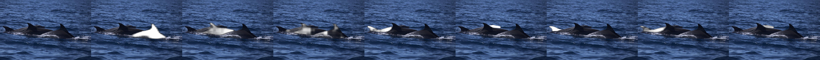IOU metric: 0.23516181851988224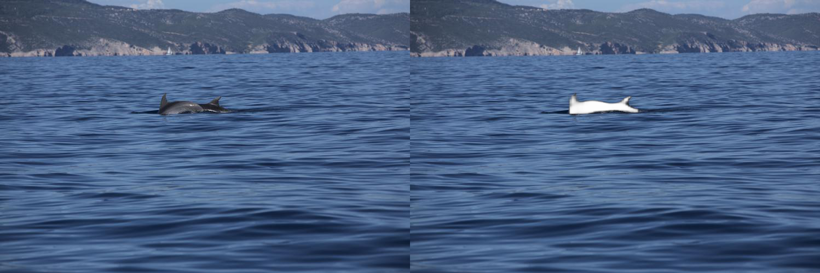IOU metric: 0.23886763657815555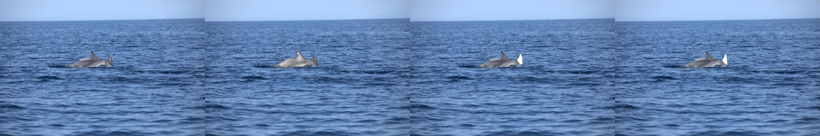IOU metric: 0.2485782288414421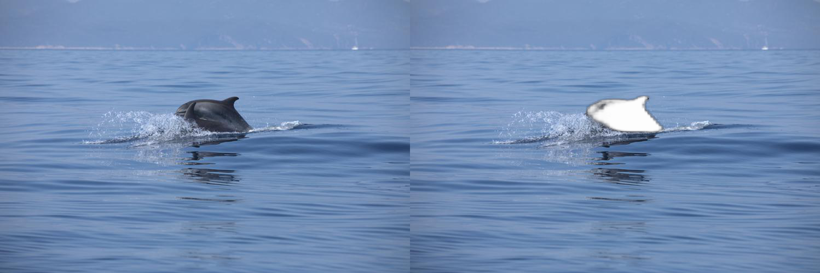IOU metric: 0.2937505354547715